Machine Learning in Radiology: How to Achieve an Accurate Diagnosis with Limited Training Data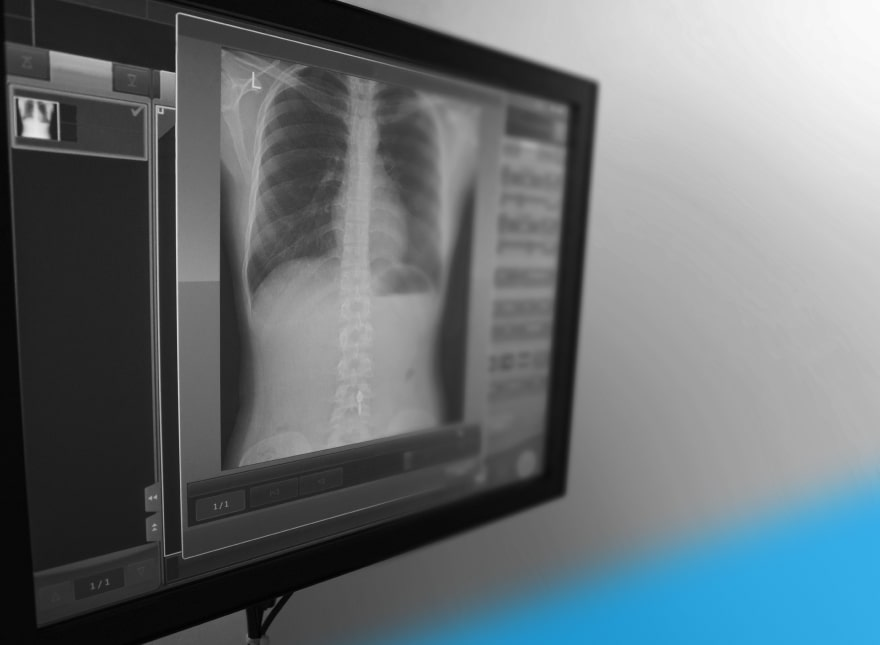Chest X-rays assist physicians in diagnosing a wide range of health conditions, including pneumonia and lung cancer. To understand how Machine Learning could facilitate the process, let us take a closer look at these X-ray images.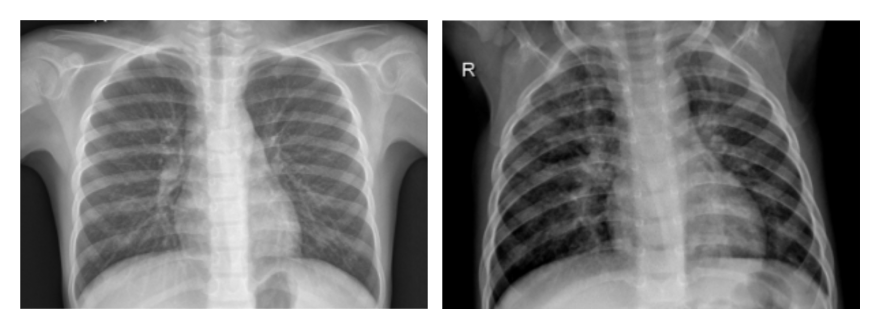On the left, you can see a chest X-ray of a healthy patient. On the right, there’s an image of a patient with confirmed pneumonia.

Physicians with no diagnostic background in radiology will have a hard time spotting swollen and inflamed areas of a patient’s lungs in those images. Even certified radiologists may confuse suspected pneumonia with other conditions—and it takes doctors approximately 20 minutes to confirm or rule out the disease.

Machine Learning models can do the job in just 10 seconds, which can be a game-changer in cases when urgent treatment is required.

## The Challenges of Applying Machine Learning Algorithms in Medical Imaging

• Insufficient dataset size. Smart medical imaging solutions feature neural networks trained on thousands of annotated X-rays. Such images might be hard to acquire due to healthcare security and patient data privacy regulations. To solve this problem, Machine Learning specialists can reuse existing image classification algorithms (transfer learning) and artificially expand the size of a dataset by modifying X-ray images (data augmentation).
• Overlapping lung disease symptoms. Developing a standalone Machine Learning model that distinguishes multiple diseases with overlapping symptoms is not a trivial case. Developers solve the problem with the help of a multi-task loss function, which estimates the accuracy of algorithms with multi-task learning (MTL) capabilities. By modifying the loss function, it is possible to simultaneously evaluate Machine Learning algorithm performance with respect to a particular image class as well as the dataset as a whole.
• Class Imbalance. In medical datasets, the images of healthy people usually outnumber X-rays indicating the presence of a disease. Although it’s the natural course of events (in the USA, only 250 thousand people seek care in a hospital due to pneumonia every year), developers need an equal amount of images to boost Machine Learning algorithm accuracy.

In this article, we will address the challenges of training medical imaging algorithms in the absence of a balanced dataset.

## Doing More with Less: How to Create Smart Medical Imaging Solutions with Imbalanced Datasets

To train Machine Learning algorithms that accurately diagnose pneumonia and determine the location and extent of the infection, developers need an equal amount of negative and positive X-rays presenting two different image classes.

The bar chart below shows the imbalance ratio in a dataset provided by the National Institute of Health (NIH) that contains radiographs of common lung conditions: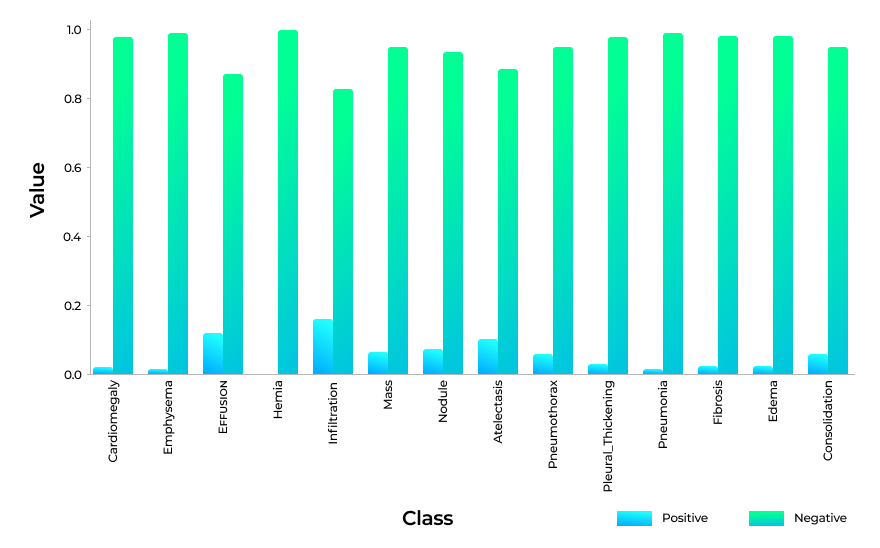It's clear from the chart that ML experts have no shortage of healthy lung X-rays, but are severely lacking X-rays of diseased lungs. Lung hernias, for example, are observed in just 0.2% of X-rays. Even pulmonary infiltrates, which can indicate pneumonia, nocardiosis, or tuberculosis, have a one-to-five ratio to negative X-ray findings.

To balance out the datasets, we can multiply each example from each class by the corresponding weight coefficient (Wpos, Wneg).

Below you will find a formula that allows us to calculate the weight coefficients, where Freqp and Freqn denote the frequency of positive and negative X-rays in the dataset:

Wpos×Freqp=Wneg×Freqn

Here’s how we can calculate the Freqp and Freqn parameters:

positive_frequencies = np.sum(labels, axis=0) / N

negative_frequencies = 1 - positive_frequencies

This technique helps eliminate the class imbalance in training datasets: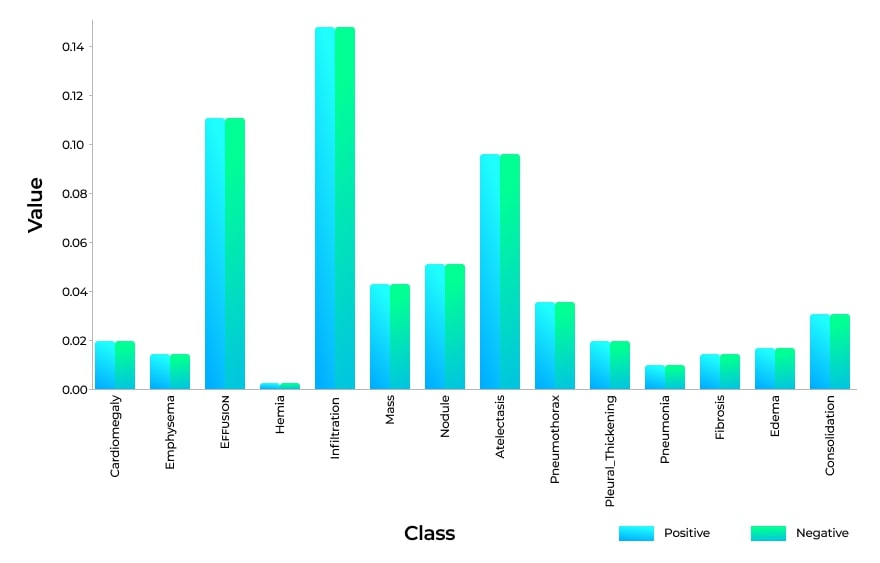Now we need to evaluate the accuracy of the Machine Learning model, and here’s where the loss function comes into play. If the model predicts results that are far from the target value, we “punish” the algorithm until it gets the predictions right.

There are different kinds of loss functions used in Machine Learning. For our purposes, we are going to use the binary cross-entropy function, which measures the performance of a classification model whose output is between zero and one.

Now we can modify the cross-entropy loss function:

Lcross-entropy(xi)= -(yi*log(f(xi))+(1-yi)*log(1-f(xi)))

Here xi and yi are the input features and the label, and f(xi) is the Machine Learning model output—i.e., the probability that a patient actually has pneumonia.

After calculating the weight coefficients, our final weighted loss for each training case will be:

Lcross-entropy(x)= -(wp*y*log(f(x))+wn*(1-y)*log(1 -f(x)))

Another way to boost the accuracy of a Machine Learning model is to resample datasets until we get an equal amount of positive and negative X-rays: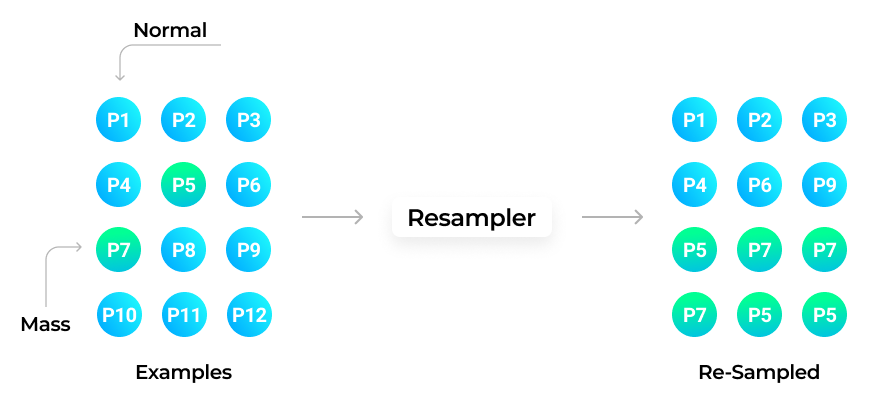To achieve the result, we combine the images and select the same quantity of negative and positive X-rays.

Now we can use the loss function without calculating the weight coefficient for the image classes. However, this approach has a considerable drawback. Since we reuse a limited number of X-rays from the same dataset, the algorithms may underperform when faced with images from a dataset other than the one they were trained on.

We will address this issue in one of our upcoming articles from the Medical Imaging series. Subscribe to our blog to have the content delivered straight to your inbox!

en